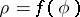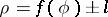# Conchoid

(diff) ← Older revision | Latest revision (diff) | Newer revision → (diff)
The planar curve obtained by increasing or decreasing the position vector of each point of a given planar curve by a segment of constant length. If the equation of the given curve isin polar coordinates, then the equation of its conchoid has the form:. Examples: the conchoid of a straight line is called the Nicomedes conchoid; the conchoid of a circle is called the Pascal limaçon.